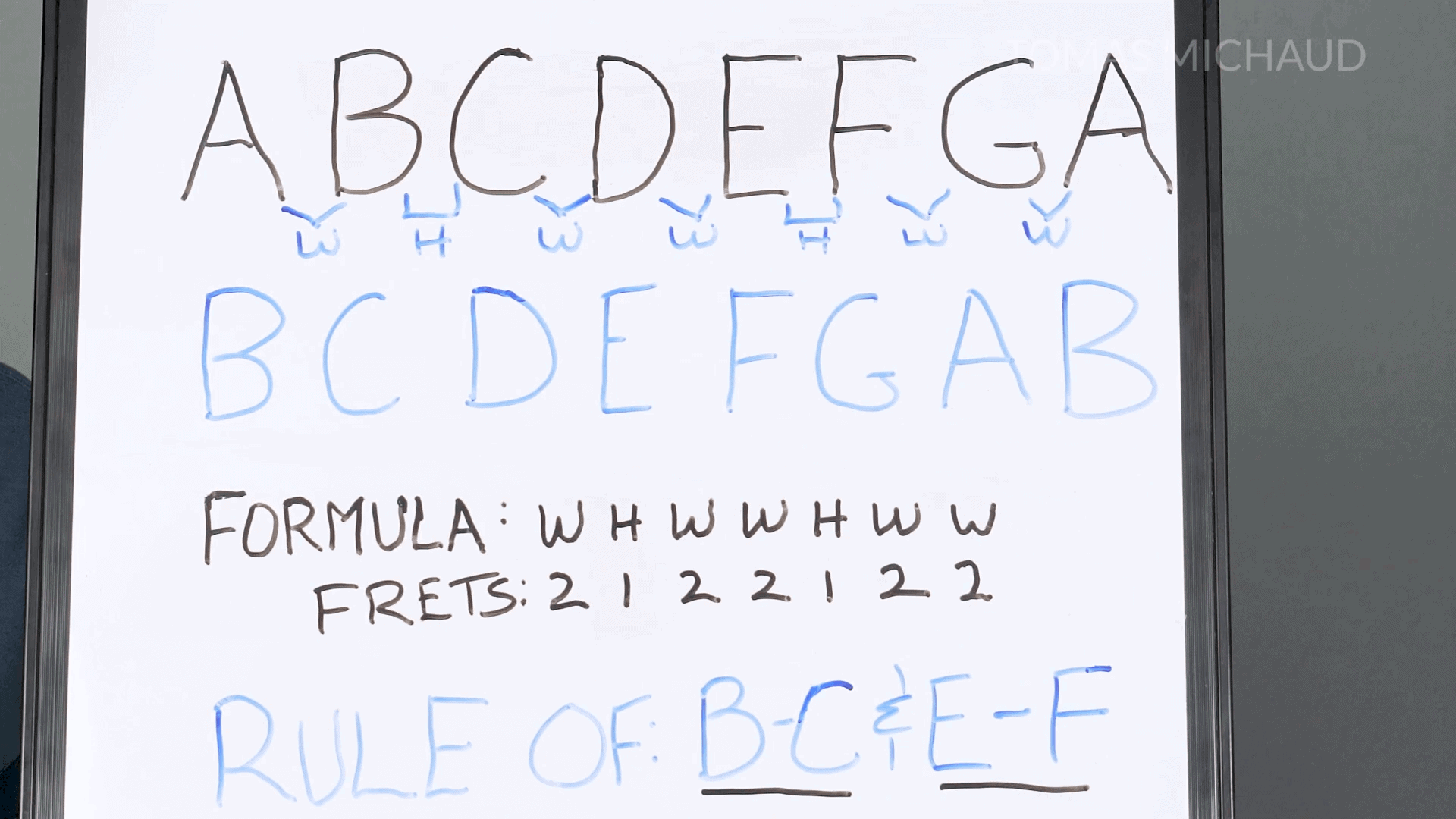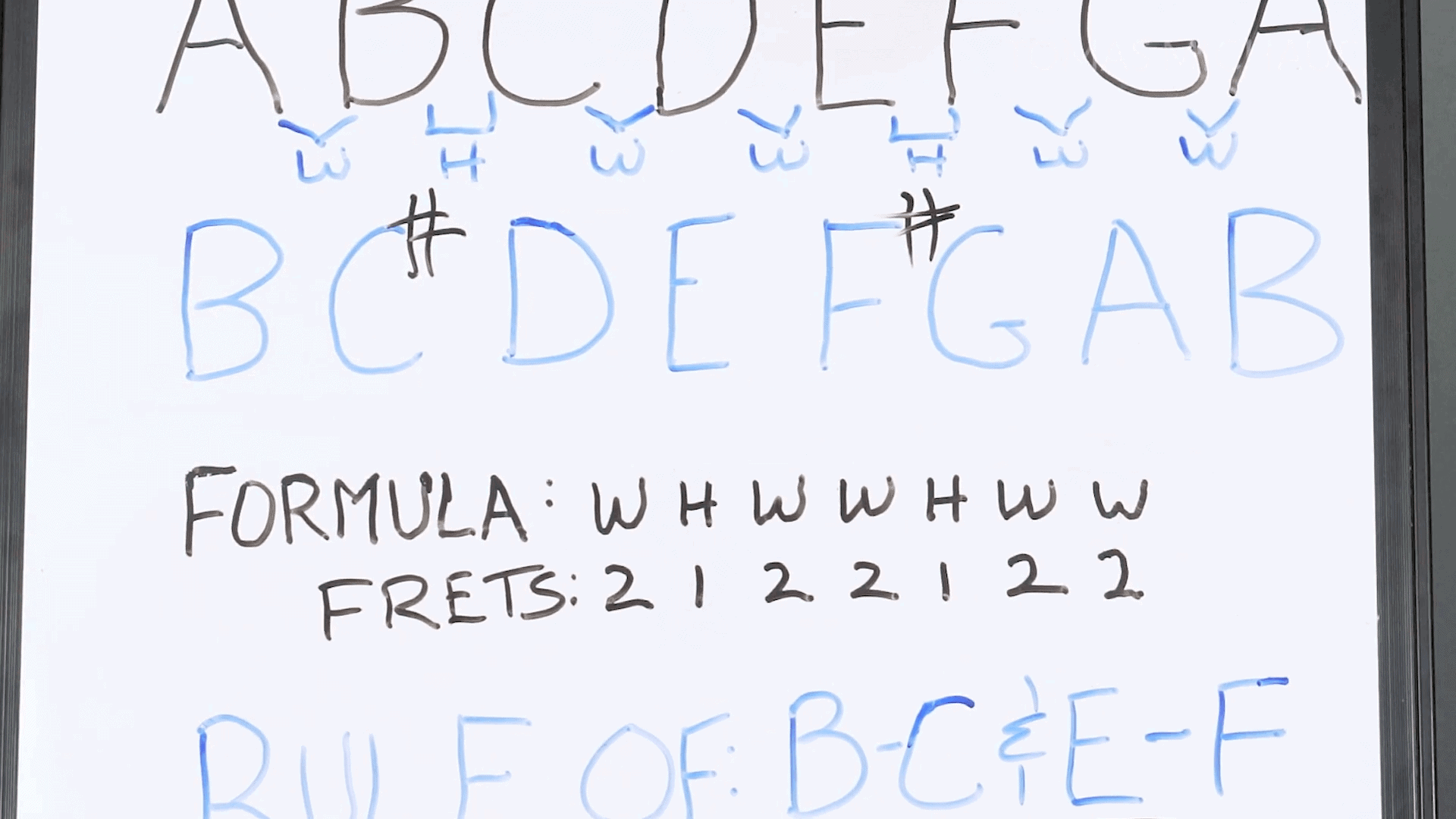# Minor Scales for Guitar Explained (in Plain English)

January 4, 2022 in Guitar Skills

What makes a minor scale sound… minor? That is exactly what we’re going to talk about in this guitar lesson. Don’t worry, you don’t have to be a music theory geek to understand this because I am going to explain it in layman's terms.

I’m going to show a formula that will produce a minor scale every time you follow it. We’ll then apply it to the guitar and see exactly how it works on the fretboard.

## What Makes The Minor Scale Minor?

The minor scale is just a series of notes that follow the following rules. We’ve got eight notes, and the scale is going to end on the same note that it starts on. In the case of the A minor scale, it will start on A and finish on A (but an octave above). For the rest of the notes we’re going to get just one of each.

The notes of the A minor scale are A, B, C, D, E, F, G, A. We can just follow the letters of the alphabet for seven straight notes and then repeat the first one.To understand the aforementioned formula clearly, let's number the notes. The first A will be 1, the B is 2, the C is 3 and so on. We call these number scale degrees. This makes it simpler to apply the formula.

For instance, the first note of the scale on any key will be 1, or the first scale degree. So on the scale of A minor, the first degree is A. And if we have D minor scale, the first degree would be D, and so on.

What’s really important is the space between the notes, also known as intervals. We use intervals to create a formula. In other words, we will apply the same distances or relationships between notes for a scale, in this case the minor scale.

## The Minor Scale Formula

The intervals for a minor scale are: from A to B one whole step. From B to C a half stp. From C to D is a whole step. From D to E another whole step. From E to F it is a half step. From F to G a whole step. Finally, from A to G we’ve got a whole step.

This delineates the minor scale formula, which can be employed in any key as long as we keep the same intervals between scale degrees. In other words, the formula for a minor scale is W H W W H W W (where “W” indicates a whole tone and “H” a half tone).

## Testing The Minor Scale Formula

Let’s test that formula to create a B minor scale. If we just follow the notes of the alphabet starting on B, we have B, C, D, E, F, G, A, B (remember its 8 notes where the first and last repeat).

Now we have to apply the formula. The first interval, or the space between the first scale degree and second scale degree (in this case B to C) is a whole step. However, from B to C we only have a half step. That means we need to raise the C a half step to to C#. This creates an interval of a whole step as we now have B to C# to conform to the minor scale formula.Next, we need a half step, which is exactly what we have from C# to D. Next up is a whole step, which is also already in place with the interval of D to E.

Then, the formula indicates we need a whole step. However E to F is just a half step, so we need to raise the F to F#, thus creating the whole step of the minor scale formula.

Next we have a half step, which is exactly what we get from F# to G. Moving on, we need a whole step, which is what we get from G to A. Finally, A to B is a whole step, fitting with the minor scale formula.

## How to Play an A Minor Scale on Guitar

Remember that on the guitar, one half step equals one fret and a whole step is two frets. Let’s try the A minor scale, by starting with the open A note (fifth string, where we will play the entire scale for now). Now we’re going to follow the formula and go up to the second scale degree with an interval of a whole step. Remember that a whole step is two half steps, so let’s go up two frets.

We’re now on the fifth string second fret. We now need a half step, so let’s move up one fret. Then we have a whole step, so let’s go up two frets, still on the fifth string.

Next, we move up a whole step or two more frets. This is followed by a half step. Next up is a whole step. Finally, we end with another whole step.

It is important that you listen closely to the notes played so you get that minor sound in your head. The distance between the notes in that formula or pattern is what makes a minor scale sound minor.

## How to Play a B Minor Scale on Guitar

Let’s apply the minor formula again to create a B minor scale on the fifth string. Let’s start with the note B on the second fret of the fifth string. From then follow the formula of whole step, half step, whole step, whole step, half step, whole step and whole step to end back on B.

Naturally, guitar players typically will play scales using more strings than just one. This creates several finger patterns to play minor scales (and other scales) that you can then move around to different keys. Naturally, we start by playing on just one string so you can better visualize the intervals involved in the minor scale formula.

## Conclusion

Learning the formula to the minor scale and practicing it will help you understand how this scale sounds and feels. This opens the doors to playing several patterns that will come in handy as you progress as a guitar player. Feel free to check out the lesson on the major scale, as it follows these same principles. Remember to go slow at first and have fun.